×#### Thank you for registering.

One of our academic counsellors will contact you within 1 working day.

Click to Chat

1800-1023-196

+91-120-4616500

CART 0

• 0

MY CART (5)

Use Coupon: CART20 and get 20% off on all online Study Material

ITEM
DETAILS
MRP
DISCOUNT
FINAL PRICE
Total Price: Rs.

There are no items in this cart.
Continue Shopping• Complete JEE Main/Advanced Course and Test Series
• OFFERED PRICE: Rs. 15,900
• View Details

```Chapter 17: Constructions Exercise – 17.3

Question: 1

Construct a △ABC in which BC = 3.6 cm, AB + AC = 4.8 cm and ∠B = 60°.

Solution:Steps of Construction:

1. Construct a line segment BC of 3.6 cm.

2. At the point B, draw ∠XBC = 60°.

3. Keeping B as center and radius 4.8cm draw an arc which intersects XB at D.

4. Join DC.

5. Draw the perpendicular bisector of DC which intersects DB at A.

6. Join AC.

Hence △ABC is the required triangle.

Question: 2

Construct a △ABC in which AB + AC = 5.6 cm, BC = 4.5 cm and ∠B = 45°.

Solution: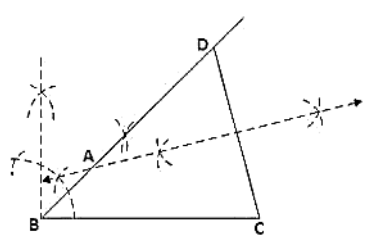Steps of Construction:

1. Construct a line segment BC of 4.5 cm.

2. At the point B, draw ∠XBC = 45°.

3. Keeping B as centre and radius 5.6cm draw an arc which intersects XB at D.

4. Join DC.

5. Draw the perpendicular bisector of DC which intersects DB at A.

6. Join AC.

Hence △ABC is the required triangle

Question: 3

Construct a △ABC in which BC = 3.4 cm, AB - AC = 1.5 cm and ∠B = 45°.

Solution:Steps of Construction:

1. Construct a line segment BC of 3.4 cm.

2. At the point B, draw ∠XBC = 45°.

3. Keeping B as centre and radius 1.5cm draw an arc which intersects XB at D.

4. Join DC.

5. Draw the perpendicular bisector of DC which intersects DB at A.

6. Join AC.

Hence △ABC is the required triangle.

Question: 4

Using rulers and compasses only, construct a △ABC, given base BC = 7 cm, ∠ABC = 60°,  AB + AC = 12 cm.

Solution: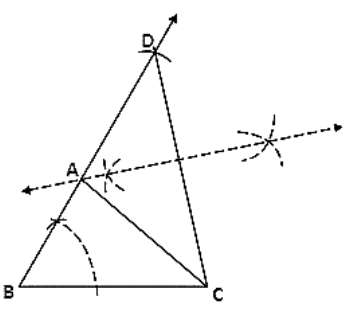Steps of Construction:

1. Construct a line segment BC of 7cm

2. At the point B, draw ∠XBC = 60°.

3. Keeping B as center and radius 12 cm draw an arc which intersects XB at D

4. Join DC

5. Draw the perpendicular bisector of DC which intersects DB at A

6. Join AC

Hence △ABC is the required triangle.

Question: 5

Construct a triangle whose perimeter is 6.4 cm, and angles at the base are 60° & 45°.

Solution: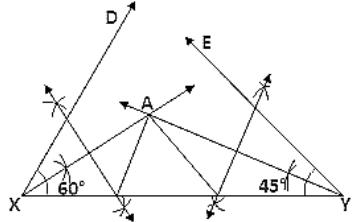Steps of Construction:

1. Draw a line segment XY of 6.4 cm.

2. Draw ∠DXY = 60° and ∠EYX = 45°.

3. Draw the angle bisectors of ∠DXY and ∠EYX which intersect each other at A.

4. Draw the perpendicular bisector of AX and AY which intersect XY at B and C respectively.

5. Join AB and AC.

Hence △ABC is the required triangle.

Question: 6

Using rulers and compasses only, construct a △ABC from the following data: AB + BC + CA = 12 cm, ∠B = 45° and ∠C = 60°

Solution:Steps of Construction:

1. Draw a line segment XY of 12 cm.

2. Draw ∠DXY = 45° and ∠EYX = 60°.

3. Draw the angle bisectors of ∠DXY and ∠EYX which intersect each other at A.

4. Draw the perpendicular bisector of AX and AY which intersect XY at B and C respectively.

5. Join AB and AC.

Hence △ABC is the required triangle.

Question: 7

Construct a right-angled triangle whose perimeter is equal to 10 cm and one acute angle equal to 60°.

Solution: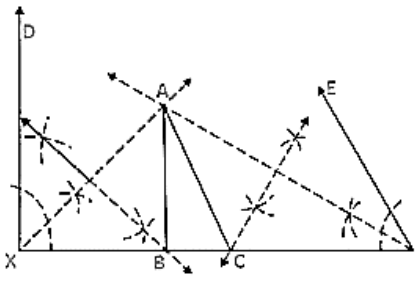Steps of Construction:

1. Draw a line segment XY of 10 cm.

2. Draw ∠DXY = 90° and ∠EYX = 60°.

3. Draw the angle bisectors of ∠DXY and ∠EYX which intersect each other at A.

4. Draw the perpendicular bisector of AX and AY which intersect XY at B and C respectively.

5. Join AB and AC.

Hence △ABC is the required triangle.

Question: 8

Construct a triangle ABC such that BC = 6 cm, AB = 6 cm and median AD = 4 cm.

Solution: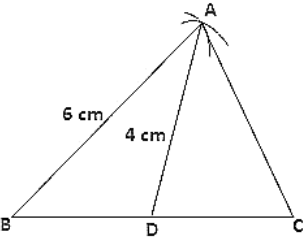Steps of construction:

1. Draw a line segment BC of 6 cm.

2. Take mid-point O of side BC.

3. With center B and D and radii 6cm and 4cm, draw two arcs which intersect each other at A.

4. Join AB, AD and AC.

Hence △ABC is the required triangle.

Question: 9

Construct a right-angled triangle ABC whose base BC is 6 cm and the sum of the hypotenuse AC and other side AB is 10 cm.

Solution: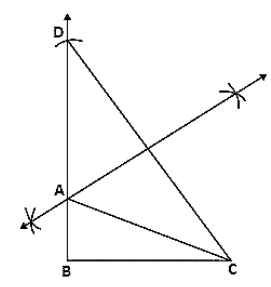Steps of Construction:

1. Construct a line segment BC of 6 cm.

2. At the point B, draw ∠XBC = 90°.

3. Keeping B as center and radius 10cm draw an arc which intersects XB at D.

4. Join DC.

5. Draw the perpendicular bisector of DC which intersects DB at A.

6. Join AC.

Hence △ABC is the required triangle.

Question: 10

Construct a triangle XYZ in which ∠Y = 30°, ∠Z = 90° and XY + YZ + ZX = 11 cm.

Solution: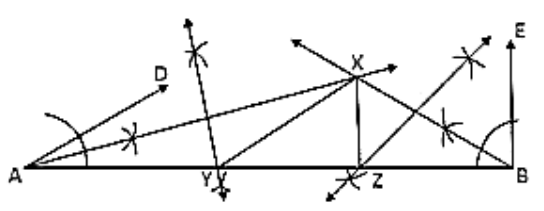Steps of construction:

1. Draw a line segment AB of 11 cm.

2. Draw ∠DAB = 30° and ∠FBA = 90°.

3. Draw the angle bisectors of ∠DAB and ∠EBA which intersect each other at X.

4. Draw the perpendicular bisector of XA and XB which intersect AB at Y and Z respectively

5. Join XY and XZ.

Hence △XYZ is the required triangle.
```### Course Features

• 728 Video Lectures
• Revision Notes
• Previous Year Papers
• Mind Map
• Study Planner
• NCERT Solutions
• Discussion Forum
• Test paper with Video Solution